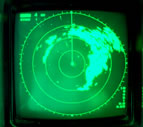×

common denominator

3 ENTRIES FOUND:
common denominator noun
plural common denominators
common denominator
noun
plural common denominators
Learner's definition of COMMON DENOMINATOR
[count]
mathematics : a number that can be divided by each of the denominators of a group of fractions
: something (such as a feature or quality) that is shared by all the members of a group of people or things